Home > CAAC > Chapter 5 > Lesson 5.2.1 > Problem5-70

5-70.
1. Find each of the following products by drawing and labeling a generic rectangle or by using the Distributive Property. Homework Help ✎

1. (x + 5)(x + 4)

2. 2y(y + 3)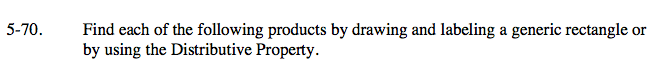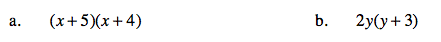Create a generic rectangle.

Put the products on the outside of the rectangle.

Find the area of the smaller rectangles.

Write the areas as a sum.
x² + 5x + 4x + 20.

(x + 5)(x + 4) = x² +9x+20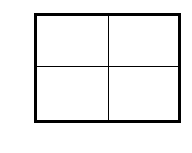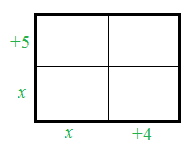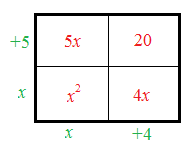Follow the steps in part (a).